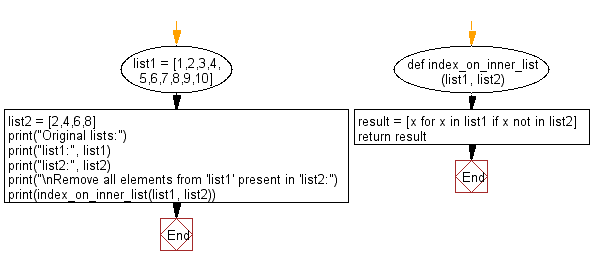﻿ Python: Remove all elements from a given list present in another list - w3resource# Python: Remove all elements from a given list present in another list

## Python List: Exercise - 117 with Solution

Write a Python program to remove all elements from a given list present in another list.

Sample Solution:

Python Code:

``````def index_on_inner_list(list1, list2):
result = [x for x in list1 if x not in list2]
return result

list1 = [1,2,3,4,5,6,7,8,9,10]
list2 = [2,4,6,8]

print("Original lists:")
print("list1:", list1)
print("list2:", list2)

print("\nRemove all elements from 'list1' present in 'list2:")
print(index_on_inner_list(list1, list2))
```
```

Sample Output:

```Original lists:
list1: [1, 2, 3, 4, 5, 6, 7, 8, 9, 10]
list2: [2, 4, 6, 8]

Remove all elements from 'list1' present in 'list2:
[1, 3, 5, 7, 9, 10]
```

Flowchart:## Visualize Python code execution:

The following tool visualize what the computer is doing step-by-step as it executes the said program:

Python Code Editor:

Have another way to solve this solution? Contribute your code (and comments) through Disqus.

What is the difficulty level of this exercise?

Test your Python skills with w3resource's quiz

﻿

## Python: Tips of the Day

Floor Division:

When we speak of division we normally mean (/) float division operator, this will give a precise result in float format with decimals.

For a rounded integer result there is (//) floor division operator in Python. Floor division will only give integer results that are round numbers.

```print(1000 // 300)
print(1000 / 300)```

Output:

```3
3.3333333333333335```